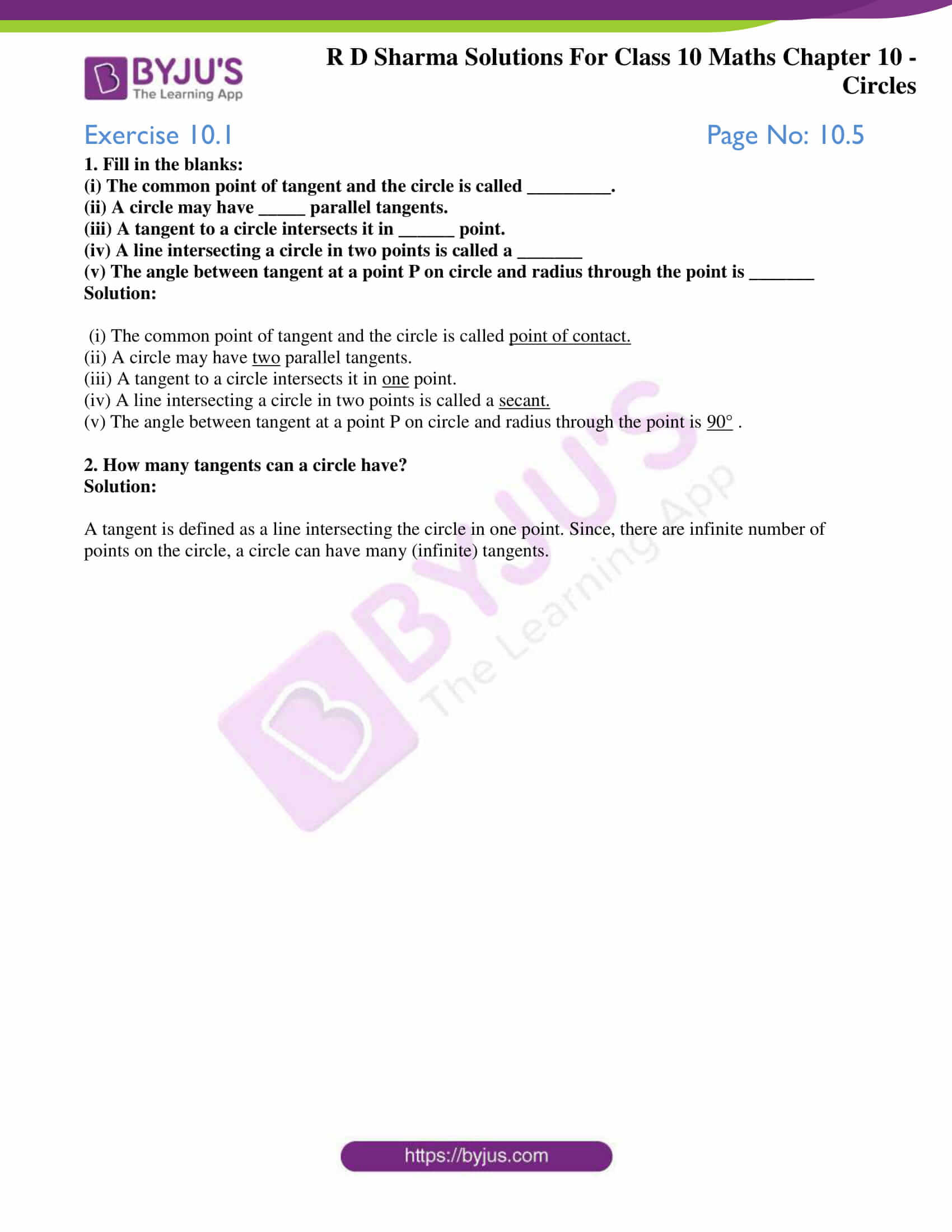# RD Sharma Solutions for Class 10 Maths Chapter 10 Circles Exercise 10.1

A Circle is just a collection of all points equidistant from a fixed point. But, it doesn’t stop there. Interesting concepts like secant and tangent to a Circle are discussed in this exercise. Students willing to build a strong foundation in these Maths chapters can access the RD Sharma Solutions Class 10. Further, students can also download RD Sharma Solutions for Class 10 Maths Chapter 10 Circles Exercise 10.1 PDF provided below for a detailed understanding of the concepts in this exercise.

## RD Sharma Solutions for Class 10 Maths Chapter 10 Circles Exercise 10.1 Download PDF### Access answers to Maths RD Sharma Solutions for Class 10 Chapter 10 Circles Exercise 10.1

1. Fill in the blanks:

(i) The common point of tangent and the circle is called _________.

(ii) A circle may have _____ parallel tangents.

(iii) A tangent to a circle intersects it in ______ point.

(iv) A line intersecting a circle in two points is called a _______

(v) The angle between tangent at a point P on circle and radius through the point is _______

Solution:

(i) The common point of tangent and the circle is called point of contact.

(ii) A circle may have two parallel tangents.

(iii) A tangent to a circle intersects it in one point.

(iv) A line intersecting a circle in two points is called a secant.

(v) The angle between tangent at a point P on circle and radius through the point is 90° .

2. How many tangents can a circle have?

Solution:

A tangent is defined as a line intersecting the circle in one point. Since, there are infinite number of points on the circle, a circle can have many (infinite) tangents.